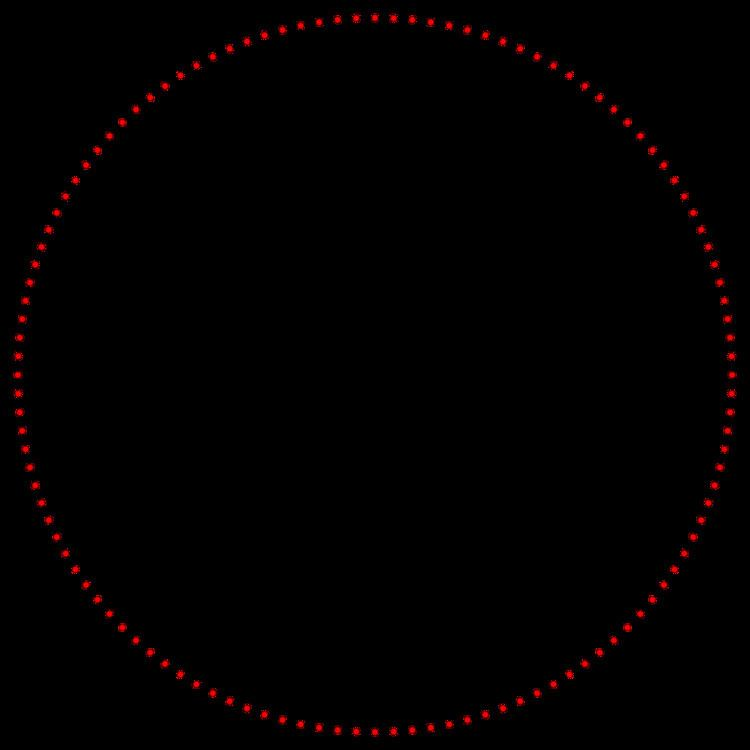# 120 gon

Updated on
Covid-19
 Type  Regular polygonInternal angle (degrees)  177° Edges and vertices  120Dual polygon  SelfSchläfli symbol  {120}, t{60}, tt{30}, ttt{15}Symmetry group  Dihedral (D120), order 2×120

In geometry, a 120-gon is a polygon with 120 sides. The sum of any 120-gon's interior angles is 21240 degrees.

## Contents

Alternative names include dodecacontagon and hecatonicosagon.

## Regular 120-gon properties

A regular 120-gon is represented by Schläfli symbol {120} and also can be constructed as a truncated hexacontagon, t{60}, or a twice-truncated triacontagon, tt{30}, or a thrice-truncated pentadecagon, ttt{15}.

One interior angle in a regular 120-gon is 177°, meaning that one exterior angle would be 3°.

The area of a regular 120-gon is (with t = edge length)

A = 30 t 2 cot π 120

and its inradius is

r = 1 2 t cot π 120

The circumradius of a regular 120-gon is

R = 1 2 t csc π 120

This means that the trigonometric functions of π/120 can be expressed in radicals.

## Constructible

Since 120 = 23 × 3 × 5, a regular 120-gon is constructible using a compass and straightedge. As a truncated hexacontagon, it can be constructed by an edge-bisection of a regular hexacontagon.

## Symmetry

The regular 120-gon has Dih120 dihedral symmetry, order 240, represented by 120 lines of reflection. Dih120 has 15 dihedral subgroups: (Dih60, Dih30, Dih15), (Dih40, Dih20, Dih10, Dih5), (Dih24, Dih12, Dih6, Dih3), and (Dih8, Dih4, Dih2, Dih1). And 16 more cyclic symmetries: (Z120, Z60, Z30, Z15), (Z40, Z20, Z10, Z5), (Z24, Z12, Z6, Z3), and (Z8, Z4, Z2,Z1), with Zn representing π/n radian rotational symmetry.

These 32 symmmetries are related to 44 distinct symmetries on the 120-gon. John Conway labels these lower symmetries with a letter and order of the symmetry follows the letter. He gives d (diagonal) with mirror lines through vertices, p with mirror lines through edges (perpendicular), i with mirror lines through both vertices and edges, and g for rotational symmetry. a1 labels no symmetry.

These lower symmetries allows degrees of freedom in defining irregular 120-gons. Only the g120 symmetry has no degrees of freedom but can seen as directed edges.

## 120-gram

A 120-gram is a 120-sided star polygon. There are 15 regular forms given by Schläfli symbols {120/7}, {120/11}, {120/13}, {120/17}, {120/19}, {120/23}, {120/29}, {120/31}, {120/37}, {120/41}, {120/43}, {120/47}, {120/49}, {120/53}, and {120/59}, as well as 44 compound star figures with the same vertex configuration.

## References

Topics

 B i Link H2
 L